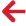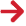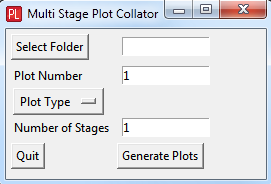﻿ PipeLay > Additional Modules > Plotting Module > Plotting Module - Automating Postprocessing

# Plotting Module - Automatic Plot CollationThe Python programming language (V2.7) was used to create a simple example script to write a plot list so that an output can be examined across multiple stages. The script, when executed, launches a small UI as shown below. This allows the user to browse to a folder containing the stage folders of interest, enter a plot number to view, select the plot type and enter the number of stages over which you require to view the plot.The verbatim Python code is given below and is also available in the Example 03 - Abandonment  directory as a Python script entitled plot_collator.pyw.

Information and links for the download and installation of Python and the associated packages used in the script are detailed in the section entitled ‘Python’.

The code comments indicated by the # symbol outline the basic operation of the script:

# Python Script for collating plots across multiple stages.
# The following steps are carried out:
#
# Libraries used are imported.
#
# Functions are defined for button presses to select the folder containing stage plots and
# to write the master plot list [*.mpl] for the plotting tool.
#
# A simple UI form is developed so the required inputs such as root directory, plot number,
# plot type & number of stages to plot over can be inputted by the user.

from Tkinter import *
import tkFileDialog
import subprocess
import os

# Set the text for the select folder button.
foldertext='Select Folder'

# Fuction for the 'Select Folder' button. You can browse to the root folder containing
# analysis stages. The button is renamed to the root folder when it is selected.
# The path to the root directory is set in folder_path
def browse():

if root_directory:
folder_button["text"] = str( os.path.split(root_directory) )
folder_path.set(root_directory)
else:
folder_button["text"] = foldertext

# Function to generate the master plot list [*.mpl] file when the 'Generate Plots'
# button is pressed.
def generate_mpl():
# Get the inputs from the UI entries
folder      = folder_path.get()
plot_type   = plot_type_option.get()
plot_number = int( plot_num_entry.get() )
num_stages  = int( num_stage_entry.get() )

# The plot name is generated from the plot type and number.
if plot_type == 'Timetrace':
plot_name = 'analysis.T' + format(plot_number,'02d') + '.mpt'
elif plot_type == 'Snapshot':
plot_name = 'analysis.S' + format(plot_number,'02d') + '.mps'
elif plot_type == 'Statistics':
plot_name = 'analysis.D' + format(plot_number,'02d') + '.mpd'
elif plot_name == 'Spectrum':
plot_name = 'analysis.F' + format(plot_number,'02d') + '.mpf'

# Open the .mpl file
f = open('stage_plot.mpl' , 'w')

# Loop over the number of required stages
# and write the path to the plot for each one
for istage in range(num_stages):
stage_num = istage + 1
stage = 'Stage ' + format(stage_num,'02d')
plot_string = folder + '/' + stage + '/' + plot_name + '\n'
plot_string = os.path.normpath(plot_string)
f.write(plot_string)

f.close()

# Call the plotting tool [PLOTTING.exe] with the *.mpl as an argument.
# Plotting tool path is hardcoded...
args =['']*2
args = r"C:\Program Files\Wood Group\PipeLay v3.4.1\bin\PLOTTING.exe"
args = r"stage_plot.mpl"

p = subprocess.Popen(args)

# SImple UI form to get inputs, write plot list and launch plotting tool
plot_form = Tk()
plot_form.geometry("260x150")
plot_form.title("Multi Stage Plot Collator")
plot_form.iconbitmap('PipeLay.ico')

# Define the variable to store the path to the stage folder
folder_path = StringVar(plot_form)
# Display the path to the stage folder
folder_box = Entry(plot_form, width=14, textvariable=folder_path)

# Entry for the plot number to collate
plot_num_entry = Entry(plot_form, width=14)
plot_num_entry.insert(END,'1')
plot_num_entry.pack()
plot_num_entry.grid(row=1, column=1)

# Plot type drop down definition
plot_type_option = StringVar(plot_form)
plot_type_option.set("Plot Type")

# Entry for the number of stages required to collate over
Label(plot_form, text="Number of Stages").grid(row=3, sticky=W, padx=5)
num_stage_entry = Entry(plot_form, width=14)
num_stage_entry.insert(END,'1')
num_stage_entry.pack()
num_stage_entry.grid(row=3, column=1)

# 'Select Folder' button to browse to root stage folder
folder_button = Button(plot_form, text=foldertext, command=browse)